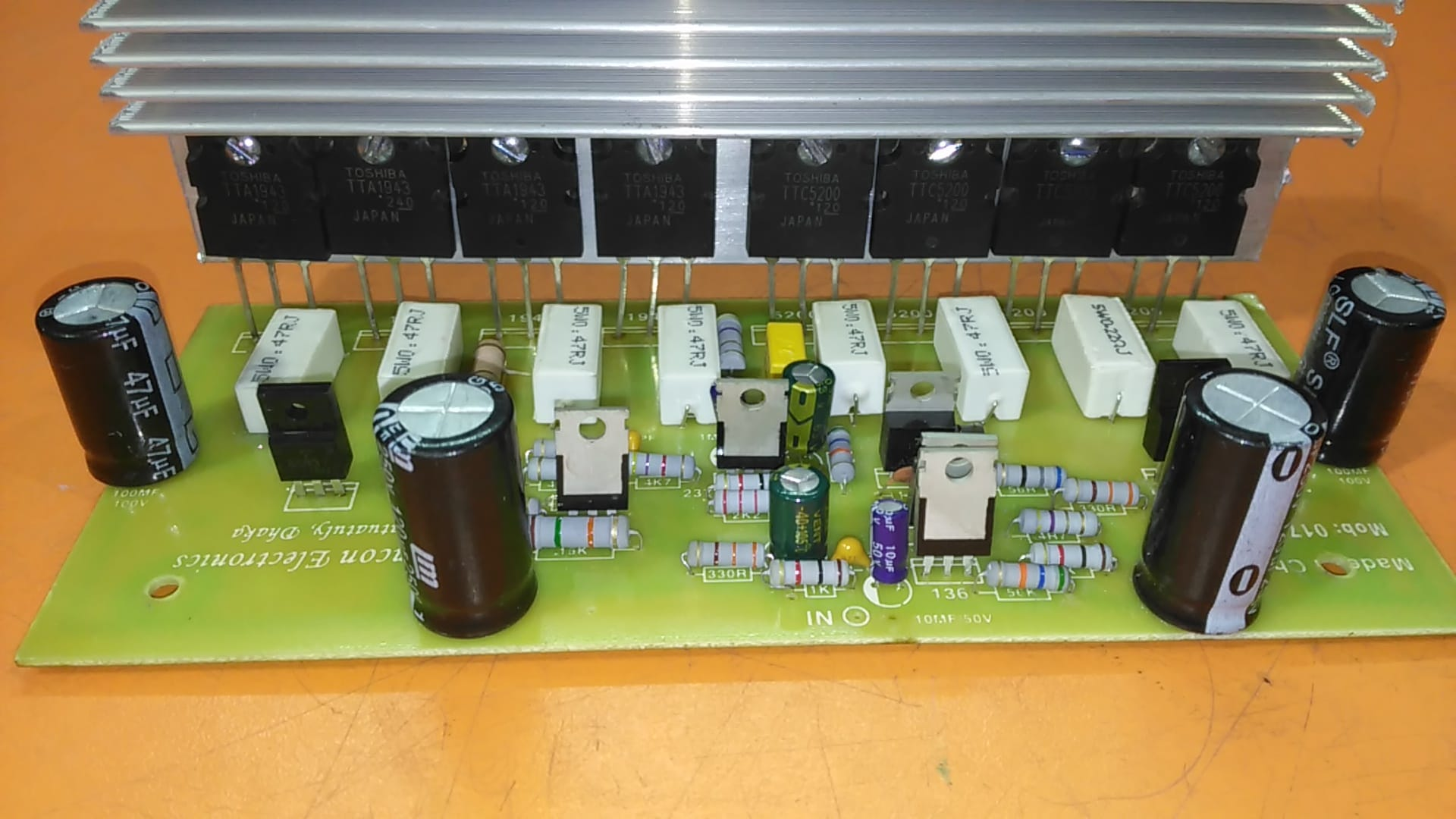# transistor amplifier tta1943 and ttc5200

## transistor amplifier tta1943 and ttc5200:

It’s very important to know about Voltage when we make a transistor amplifier. Voltage is important to an amplifier. Every Audio IC or audio transistor have there own rules for using voltage. this why we have to follow the rules. if we use voltage without following the rules we will lose the IC or transistor. here in this video, we can see how to use voltage according to the transistor. how many transistors we are using. We know that one transistor is 1.2 amperes. and we can use the maximum 70 voltage according to the circuit diagram. Watts depend on Voltage and Ampere of the transformer we are using.how many transistors we have to use it will depend on our transformer watts. for an example, we will make a 500 Watts amplifier. So we need a 500 watts transformer. our transformer is 50-0-50 Voltage and 10 amperes. we know that Voltage X ampere = Watts. how many transistors need? it will depend on ampere. Always we have to follow the rules.

Let’s watch the video,

Now we have to calculate how many transistors we have to use? we know that one transistor can take a maximum of 1.2 amperes. 1.2 X 8 =9.6 ampere. so we have to use a minimum of 8 transistors. but we will use 12 transistors for good performance. another example, If we need an 800 Watts amplifier then what to do?

transistor circuit diagram of 2sa1943 and 2sc5200

Thanks a lot to be with us. If you want another post then please visit our website.

We have another post for you. like repairing amplifier,

Please like comment and share our post.